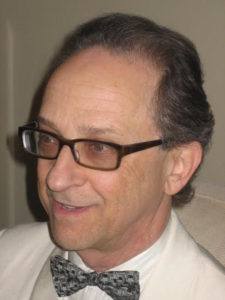## Posts Tagged ‘guest post’

### Solving the Measurement Problem (Guest Post)

Wednesday, October 5th, 2016

The following is a guest posting from Ken Krechmer of the College of Engineering at Applied Science at the University of Colorado, at Boulder.Ken Krechmer

The dichotomy between quantum measurement theory and classical measurement results has been termed: the measurement disturbance, measurement collapse and the measurement problem.   Experimentally it is observed that the measurement of the position of one particle changes the momentum of the same particle instantaneously.  This is described as the measurement disturbance.  Quantum measurement theory calculates the probability of a measurement result but does not calculate an actual measurement result.  What occurs that causes the quantum measurement probability to collapse into a classical measurement result?  Different approaches have been proposed to resolve one or both of these issues including hidden variables, non-local variables and decoherence, but none of these approaches appear to fully resolve both these aspects of the measurement problem.

Further complicating this measurement problem: 1. The quantum effect called entanglement is another measurement disturbance where the measurement of one particle instantaneously impacts a similar measurement of another, far remote, particle.  2. The quantum effect called uncertainty which defines the minimum variation between two measurement results and changes depending on the order of the two measurements.

Relational measurements and uncertainty,” also available at Measurement, resolves both aspects of the measurement problem by expanding the definition of a classical measurement to include sampling and calibration to a reference. Experimentally, it is well known that a measurement must be sampled and calibrated to a reference to establish a measurement result. This paper proves that the measurement collapse is due to the effect of sampling and calibration which is equal to the universal quantum measurement uncertainty.  The universal quantum measurement uncertainty has been verified in independent quantum experiments. Next, one quantum measurement is shown to instantaneously disturb another because one sampling and calibration process is applied to both measurement results.

The paper resolves the dichotomy between quantum theory and classical measurement results, derives the quantum uncertainty relations using classical physics, unifies the measurement process across all scales and formally models calibration and sampling.